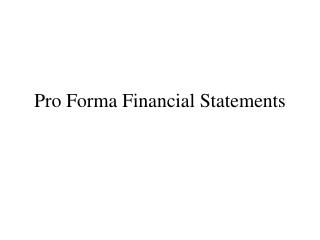DownloadDownload PresentationPro Forma Financial Statements

# Pro Forma Financial Statements

Download Presentation## Pro Forma Financial Statements

- - - - - - - - - - - - - - - - - - - - - - - - - - - E N D - - - - - - - - - - - - - - - - - - - - - - - - - - -
##### Presentation Transcript

1. Pro Forma Financial Statements

2. Pro Forma Financial Statements • Projected or future financial statements. • Pro forma income statements, balance sheets, and the resulting cash flow statements are the building blocks of financial planning. • They are also vital for any valuation exercises one might do in investment or M&A planning.

3. Generic Forms: Income Statement Sales (or revenue) Less Cost of Goods Sold Equals Gross Income (or Gross Earnings) Less Operating Expenses Equals Operating Income Less Depreciation Equals EBIT Less Interest Expense Equals EBT Less Taxes Equals Net Income (EAT)

4. Assets Cash Accounts Receivable Inventory Marketable Securities Total Current Assets Gross PP&E Accumulated Depreciation Net PP&E Land Total Assets Liabilities + Equity Bank Loan Accounts Payable Wages Payable Taxes Payable Current Portion – L-T Debt Total Current Liabilities Long-Term Debt Preferred Stock Common Stock Retained Earnings Total Liabilities + Equity Generic Forms: Balance Sheet

5. Generic Forms: Bridge • Clearly we can’t hope to get anywhere if we treat these forecasts as being separate. The income statement records the effect of a given year while the balance sheets show the situation at the beginning of and after that year. • One important bridge is: Net Income less Dividends = Change in Retained Earnings • This simple relation and interest expense will tie them together.

6. Bridge? Income Statement Balance Sheet

7. Overview of the Process • The most common way to proceed is to fill in the income statement first. The standard approach is called “percent of sales forecasting.” • Because you first get the sales or sales growth forecast. • Then, project variables having a stable relation to sales using forecasted sales and estimated ratios. • COGS, Inventory, Operating expenses (may) • D&A, Interest expense (won’t), Taxes (will be predictable from EBT) • Require estimates of the components of expenses that don’t vary directly (and in a stable way) with sales to complete the income statement.

8. Overview of the Process… • From the completed income statement, determine the change in retained earnings, transfer it to the balance sheet. • Some of the current assets and liabilities (accounts receivable, accounts payable, inventory, wages payable, etc.) can be expected to vary directly with sales. • Cash is usually determined by a policy decision via some inventory (of liquidity) model. • Gross PP&E changes are usually the result of policy decisions as are changes in preferred or common stock or long-term debt. • Often the bank loan or long-term debt is used as a residual to determine the required new financing (make it balance).

9. Overview of the Process • Interest expense comes from the amount of interest bearing (Long-term) debt. • Interest expense effects net income, • Which effects changes in retained earnings, • Which, through the equality requirement for the balance sheet, effects the amount of interest bearing debt that is necessary. • Thus these two statements are intimately connected.

10. Circularity

11. Interactions… • The income statement equation can be written: [Rev – Operating Exp – Depr&Amort - (Int Bearing Debt)(Int Rate)](1- Tax Rate) - Dividends = Change in retained earnings • The balance sheet equation is: Total Assets = Accts Pay + Wages Pay + Taxes Pay + Int Bearing Debt + Common Stock + Change in retained earnings • Interest bearing debt is the unknown in each equation. • If we just substitute the LHS of the income statement equation for the last term of the balance sheet equation we can “solve them simultaneously” to find the external debt financing required. • This is made easy by spread sheets and should be easier to understand by looking at the following example.

12. Example